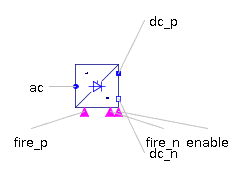Singlephase Electromagnetic Converter - MapleSim Help

Home : Support : Online Help : MapleSim : MapleSim Component Library : Magnetic : Fundamental Wave : Components : Singlephase Electromagnetic Converter

Fundamental Wave Single Phase Electro Magnetic Converter

Single Phase Electromagnetic ConverterDescription The Fundamental Wave Single Phase Electromagnetic Converter performs a lossless conversion between the single-phase electric domain and the fundamental-wave magnetic domain.Equations ${\stackrel{^}{V}}_{m}={\stackrel{^}{V}}_{{m}_{p}}-{\stackrel{^}{V}}_{{m}_{n}}=\frac{2}{\mathrm{\pi }}Ni$ $\stackrel{^}{\mathrm{\Phi }}={\stackrel{^}{\mathrm{\Phi }}}_{p}=-{\stackrel{^}{\mathrm{\Phi }}}_{n}$ $i={i}_{p}=-{i}_{n}$ $v={v}_{p}-{v}_{n}=-\Re \left(\stackrel{&conjugate0;}{N}\stackrel{^}{\mathrm{\Phi }}\right)$ $N={n}_{\mathrm{eff}}\mathrm{exp}\left(j\mathrm{\theta }\right)$Variables

 Name Units Description Modelica ID $v$ $V$ Voltage drop v $i$ $A$ Current i ${\stackrel{^}{V}}_{m}$ $\frac{1}{A}$ Complex magnetic potential difference V_m $\stackrel{^}{\mathrm{\Phi }}$ $\mathrm{Wb}$ Complex magnetic flux PhiConnections

 Name Description Modelica ID ${\mathrm{pin}}_{p}$ Positive pin pin_p ${\mathrm{pin}}_{n}$ Negative pin pin_n ${\mathrm{port}}_{p}$ Positive complex magnetic port port_p ${\mathrm{port}}_{n}$ Negative complex magnetic port port_nParameters

 Name Default Units Description Modelica ID ${n}_{\mathrm{eff}}$ $1$ Effective number of turns effectiveTurns $\mathrm{\theta }$ $\mathrm{rad}$ Orientation of the resulting fundamental wave phasor orientationModelica Standard Library The component described in this topic is from the Modelica Standard Library. To view the original documentation, which includes author and copyright information, click here.